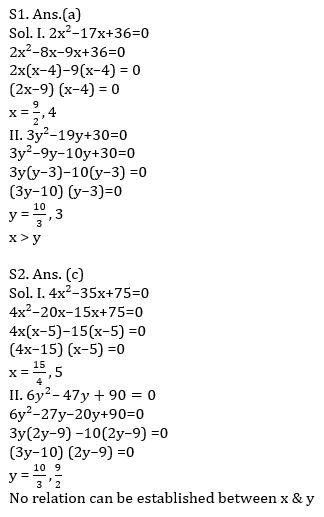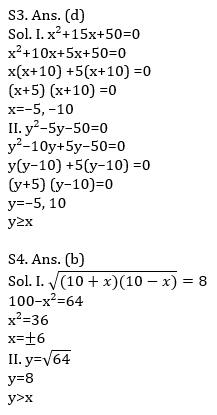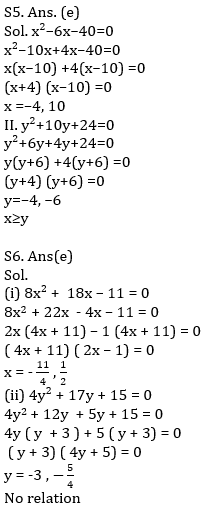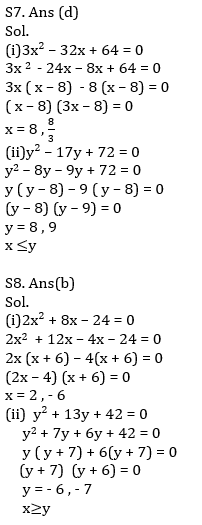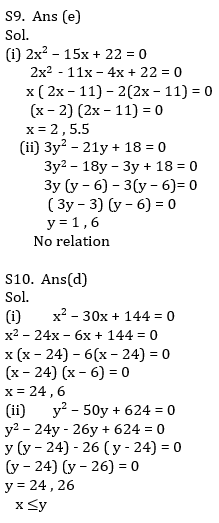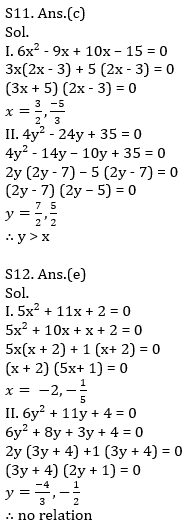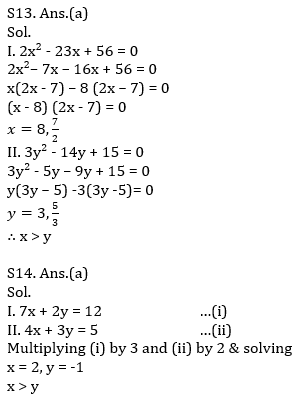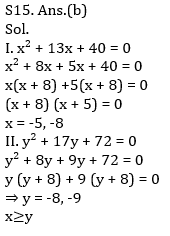Latest Banking jobs   »   Quantitative Aptitude Quiz For IBPS RRB...

# Quantitative Aptitude Quiz For IBPS RRB PO, Clerk Prelims 2021- 3rd June

Directions (1-5): In each of these questions, two equations (I) and (II) are given. You have to solve both the equations and give answer
(a) if x>y
(b) if x<y
(c) if x = y or no relation can be established between x and y
(d) if x ≤y
(e) if x≥y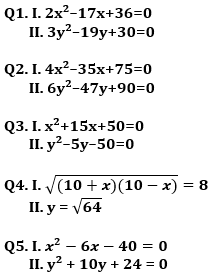Directions (6-10): In each of these questions, two equations (I) and (II) are given. You have to solve both the equations and give answer
(a) if x>y
(b) if x≥y
(c) if x<y
(d) if x ≤y
(e) if x = y or no relation can be established between x and y.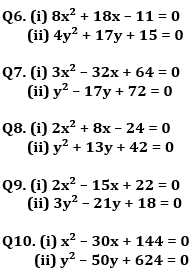Directions (11-15): In each of these questions, two equations (I) and (II) are given. You have to solve both the equations and give answer.
(a) if x>y
(b) if x≥y
(c) if x<y
(d) if x ≤y
(e) if x = y or no relation can be established between x and y.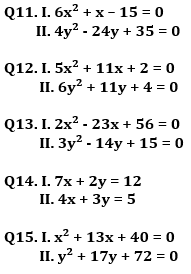Practice More Questions of Quantitative Aptitude for Competitive Exams:

###### Study Plan for IBPS RRB PO/Clerk Prelims 2021

Solutions